Courses

# Phase Equilibria (Part - 2)- Thermodynamic and Statistical Physics, CSIR-NET Physical Sciences Physics Notes | EduRev

## Physics for IIT JAM, UGC - NET, CSIR NET

Created by: Akhilesh Thakur

## Physics : Phase Equilibria (Part - 2)- Thermodynamic and Statistical Physics, CSIR-NET Physical Sciences Physics Notes | EduRev

The document Phase Equilibria (Part - 2)- Thermodynamic and Statistical Physics, CSIR-NET Physical Sciences Physics Notes | EduRev is a part of the Physics Course Physics for IIT JAM, UGC - NET, CSIR NET.
All you need of Physics at this link: Physics

II. A system at constant volume and temperature

Since we now know that at equilibrium there is only one temperature defined for the two phases let us now remove the system from isolation and place it in a heat bath at temperature, T. We will still hold the volume constant. Under conditions of constant temperature and volume we know that the criterion for equilibrium is that the Helmholtz free energy seeks a minimum. That is,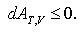Our system now looks like,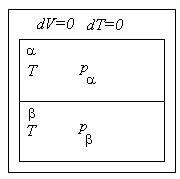but we do not know the relationship between the pressures in the two phases.

Let us now transfer a small amount of volume, dV > 0, from phase α to phase β .

We know that dA = − SdT − pdV = − pdV for a constant temperature system. So,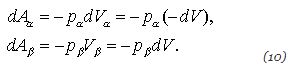The change in Helmholtz free energy for the entire system is,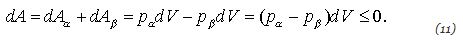Since dV is positive we conclude that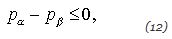or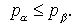If the system is not at equilibrium then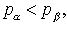which makes sense since the β phase expanded at the expense of the α phase.

If the system is at equilibrium then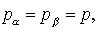so that there is only one pressure defined for the system.

III. The system at constant pressure and temperature

Since we now know that at equilibrium both phases are at the same temperature and pressure, let us remove the constant volume restriction and place our system in a heat bath at temperature, T, and a pressure bath at pressure, p. (The atmosphere is a good example of a pressure bath at approximately one atmosphere pressure. You can make chambers to hold different pressures if you wish.) Our system looks like,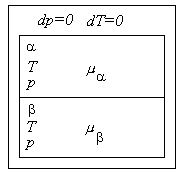but we do not know the relationship between the chemical potentials in the two phases.

At constant temperature and pressure we know that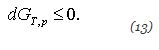We also know that,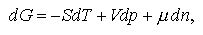which at constant temperature and pressure becomes,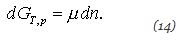Let us now move a small amount of material, dn, from phase α to phase β (with dn > 0). Then,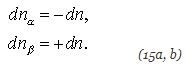and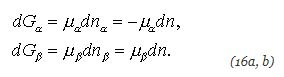The change in Gibbs free energy for the entire system is then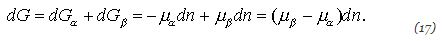Since dn is positive by construction we conclude that,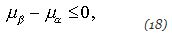or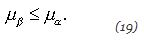If the system is not in equilibrium then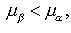which makes sense since material wants to move from a region of higher chemical potential to a region of lower chemical potential.

If the system is in equilibrium then,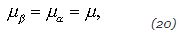so that there is only one chemical potential defined for the system.

Our conclusion, then, is that two phases in equilibrium must have the same temperature, pressure and chemical potential. The above sequence of derivations can easily be extended to include more phases and/or extended to include mixtures, where we would find that the temperature, pressure, and the chemical potential of each component must be the same in every phase. We will use these facts a little later in our derivation of the Gibbs phase rule.

159 docs

,

,

,

,

,

,

,

,

,

,

,

,

,

,

,

,

,

,

,

,

,

,

,

,

;